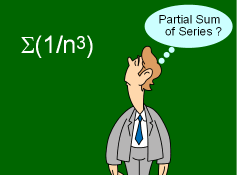Ch 12. Infinite Sequences and Series Multimedia Engineering Math Sequencesand Series Integral andComparison Alt. Series &Abs. Conv. PowerSeries Taylor, Mac. &Binomial
 Chapter 1. Limits 2. Derivatives I 3. Derivatives II 4. Mean Value 5. Curve Sketching 6. Integrals 7. Inverse Functions 8. Integration Tech. 9. Integrate App. 10. Parametric Eqs. 11. Polar Coord. 12. Series Appendix Basic Math Units Search eBooks Dynamics Fluids Math Mechanics Statics Thermodynamics Author(s): Hengzhong Wen Chean Chin Ngo Meirong Huang Kurt Gramoll ©Kurt GramollMATHEMATICS - CASE STUDY IntroductionProblem Diagram In order for Peter to be admitted into the doctoral program at the School of Mathematics, he has to pass the qualifying exam. During the oral portion of the exam, Peter is struggling to recall how to obtain the partial sum of a series using the integral test concept. Could you help him out? Questions Estimate the sum of the series Σ(1/n3) using the partial sum of the first five terms. What is the error involved? Approach Approximate the sum using the midpoint of the lower and upper bound for S given by the inequalities presented in the theory section.